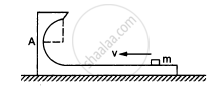Department of Pre-University Education, KarnatakaPUC Karnataka Science Class 11
Advertisement Remove all ads

# Figure shows a small block of mass m which is started with a speed v on the horizontal part of the bigger block of mass M placed on a horizontal floor. - Physics

Sum

Figure shows a small block of mass m which is started with a speed v on the horizontal part of the bigger block of mass M placed on a horizontal floor. The curved part of the surface shown in semicircular. All the surfaces are frictionless. Find the speed of the bigger block when the smaller block reaches the point A of the surface.Advertisement Remove all ads

#### Solution

The mass of the small block is m.
Initial speed of this block is v.

The mass of the bigger block is M.
Initial speed of this block is zero.

At point A, as the small block transfers its momentum to the bigger block, both the blocks move with a common velocity V (say) in the same direction as v.

Using the law of conservation of linear momentum, we can write:
Initial momentum = final momentum

MV + M × O = ( m +M)V

⇒ V = (mv)/(m + M)

Therefore, the speed of the bigger block when the smaller block reaches point A of the surface is (mv)/(m + M).

Is there an error in this question or solution?
Advertisement Remove all ads

#### APPEARS IN

HC Verma Class 11, 12 Concepts of Physics 1
Chapter 9 Centre of Mass, Linear Momentum, Collision
Q 29 | Page 161
Advertisement Remove all ads
Advertisement Remove all ads
Share
Notifications

View all notifications

Forgot password?